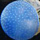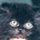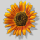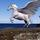## General Question# Could you explain this simple maths problem?

Asked by hjfffdfhfk (3) June 27th, 2009

http://www.bbc.co.uk/schools/gcsebitesize/maths/images/figure_3.gif
I need to work out PS, could you show me what to do and explain why you did what you did on each step?

Observing members: 0Composing members: 0Well, I’m missing the fact whether these two rectangles are similar or not. If they are, you can compare the sides. 4/7 = 9/x -> 7/4 = x / 9 —> 7*9 / 4 = x—> x = 15.75

LanceVance (645)“Great Answer” (1) Flag as…or 9/4 =2.25
2.25×7 = 15.75

In the first rectangle, if you compare the length of PS to QP, the proportionality is 9/4 or 2.25. You have the value of QP in the second rectangle, and if they are proportional, then multiplying the known value of QP in the second rectangle will give you the value of PS.

PandoraBoxx (18026)“Great Answer” (0) Flag as…PS/9 = 7/4 so just to make sure on this that means the fraction of PS over 9 equals the same as 7 over 4

Then times both sides by 9:
PS = 7/4 * 9 ok why are we timing it by 9, are we only timing the 7/4 if so why?

(7*9)/4 = 63/4 = 15.75 why is it 7 times 9 and then /4? and why do they / between 4 again?

hjfffdfhfk (3)“Great Answer” (0) Flag as…9/4 = PS/7
PS1/QP1 = PS2/QP2

PandoraBoxx (18026)“Great Answer” (0) Flag as…@PandoraBoxx
?what? I don’t really care on the answer only how do I get to it and why?

hjfffdfhfk (3)“Great Answer” (0) Flag as…If the two rectangles are proportional, then the comparison between QP and PS stays the same. PS is always 2.25 times bigger than QP.

PandoraBoxx (18026)“Great Answer” (0) Flag as…edit

hjfffdfhfk (3)“Great Answer” (0) Flag as…It should be 15.75 if the two are proportional.
Side 7 is to side 4, as side PS is to side 9.
Side 7 is 7/4 or 1.75 times longer than side 4.
Therefore, side PS is 1.75 times longer than 9 which is 15.75.

@LanceVance & @PandoraBoxx did it correctly, as well. We’ve shown you three different ways to the same answer.

whatthefluther (13820)“Great Answer” (0) Flag as…To sum up, there are two ways of looking at the problem:

1. In similar figures the ratio of two sides in one is the same as the ratio of the corresponding sides in the other.
PS:7=9:4 or PS/7 = 9/4

2. If two figures are similar, one of them may be viewed as an enlargement of the other, so that the ratio of corresponding sides will be the same.
PS:9 = 7:4 or PS/9 = 7/4

Another way of saying this is that everything in the larger rectangle is 7/4 or 1.75 as large as the corresponding side in the smaller rectangle. That is to say that the scaling factor is 1.75.

What is nice about this viewpoint is that if you had two similar figures with many sides of different lengths, you could get from one to the other by multiplying or dividing the length by the scaling factor. Additionally, if you knew the area of one you could get the area of the other by multiplying or dividing by the square of the scaling factor.

LostInParadise (31275)“Great Answer” (1) Flag as…@whatthefluther
I’ll say again I don’t care about the answer, how do you work out 2.25? Id rather do it the other way but I don’t understand why we have to do this (7*9)/4 = 63/4

hjfffdfhfk (3)“Great Answer” (0) Flag as…You do seem to be having trouble with this. Let me try again.

Suppose that the two short sides of the rectangles were 8 cm and 4 cm instead of 7 cm and 4cm. That would mean that the rectangle on the right is larger by a factor of 8/4=2 and the large side of the rectangle would be 2*9=18 cm.

In the actual problem we have an enlargement factor of 7/4=1.75 so the larger rectangle is bigger by a factor of 1.75. 1.75 * 9 = 17.75 cm.

LostInParadise (31275)“Great Answer” (0) Flag as…If anyone is still following this, that should be 15.75 cm for the answer, as was discreetly pointed out to me in a PM.

LostInParadise (31275)“Great Answer” (0) Flag as…or